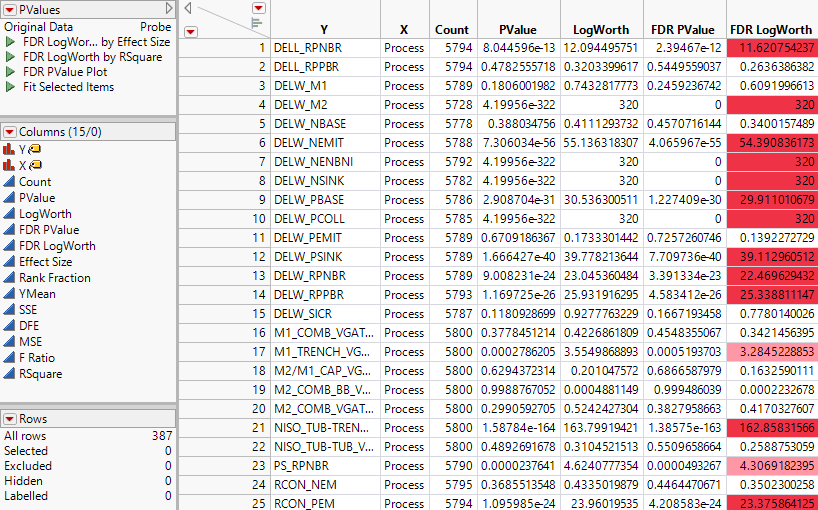Publication date: 07/30/2020

# The PValues Data Table

The PValues data table contains a row for each pair of Y and X variables. If you specified a column for Group, the PValues data table contains a first column called Group. A row appears for each level of the Group column and for each pair of Y and X variables. The PValues data table also contains a table variable called Original Data that gives the name of the data table that was used for the analysis. If you specified a By variable, JMP creates a PValues table for each level of the By variable, and the Original Data variable gives the By variable and its level.

Figure 21.7 shows the PValues data table created in Example of Response Screening.

Figure 21.7 PValues Data Table, Partial View